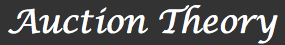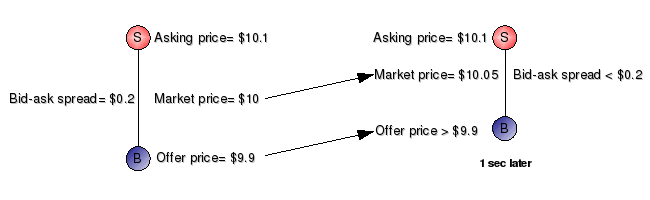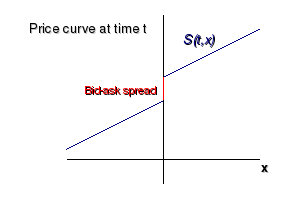Introduction1. Why study auctions? 2. The English and second-price sealed bid auctions 3. The Dutch and first-price  sealed bid auctions 4. The Revenue Equivalence  Theorem and further readingWhy study auctions?

## Price discovery

Market models in financial mathematics often assume that buyers and sellers in the market act as "price takers". This assumption is reasonable, for example, when the number of buyers and sellers is big enough so that nobody believes his actions will influence prices. This hypothesis is known as the Competitive market hypothesis. There exist however many examples when this assumption does not hold and it becomes crucial to explain where prices come from and the influence of players' actions on them. Auction theory provides one explicit model of price making (ignoring bargaining aspects of the process).Regarding the Competitive market hypothesis, it is important to observe that most of the theory of Mathematical Finance has been developed by assuming this hypothesis to hold. When this assumption is dropped the models become more complex. One way to approach non-competitive markets is by specifying in the model, the price of the assets as a function not only of time but also of the size of order. In other words for every asset in the market we would have a family of price processes, S(t,x) that model, from the perspective of a large investor, the price of the asset at time t if x shares of the asset are bought (x>0) or sold (x < 0). There are many assumptions that have to be made in order to get nice results. The most important one of them is the fact that as x increases the price S(t,x) should either remain unchanged or increase as well (see figure).This accounts for the fact that if a large number of shares have to be bought, since there is a limited ammount of cheap shares, the investor will have to pay more on average. It excludes however, a phenomenon often seen in consumer products when buyers get discounts for purchasing large quantities of a product.

## Auction market

A more practical reason for studying auctions is that the value and diversity of goods exchanged in the auction market is huge. For instance in 2007 the total revenue from goods and services sold at live auction was \$270.7 billion. Among the many goods exchanged in auctions, excluding online auctions, are: antiques, collectibles, wines, real estate property, commodities, US Treasury bills, etc. Online auction companies have grown in number during the past years. Among them we find eBay, Amazon.com, Yahoo, etc. Therefore, the size of the auction market highlights the importance of studying Auction Theory.

## The Revelation Principle

From the game-theoretical point of view auctions are games with incomplete information or Bayesian games: the player's payoff functions are not common knowledge. This characteristic is due to the fact that in an auction, bidders are uncertain about other players' valuation of the item or good, and sometimes are even uncertain about their own valuation. A quite useful result in game theory, states that any Bayesian Nash equilibrium in a Bayesian game (no matter the nature of the game) can be represented as a Bayesian Nash equilibrium of a particular type of auction in which:
1. The bidders simultaneously make (possibly dishonest) claims about their valuations. Say, that there are N bidders with valuations and claims v1,...,vN and c1,...,cN, respectively.
2. Given the bidders' claims about their valuations each player i pays xi(c1,...,cN) and receives the good with probability qi(c1,...,cN). The sum q1(c1,...,cN)+...+qN(c1,...,cN) must be less than or equal to 1.
3. Telling the truth is a Bayesian Nash equilibrium for each player.

An auction with the properties 1.-3. is known as an incentive-compatible direct mechanism. This result is known as The Revelation Principle, an important tool for designing games with private information and studying auction and bilateral trading problems. This theorem simplifies the study of auctions itself, but at the same time explains why understanding such mechanisms may be of great importance in order to understand the behavior of other institutions.

## Subsequent hypotheses

Before going ahead and studying particular examples of auctions, it is important to state a precise definition of the concept and list the assumptions we will make in the subsequent lessons. By relaxing or completely changing some of these assumptions new results are obtained. The study of more general models is out of the scope of these lessons, however in lesson 4 we mention some results related to extensions of the model.

An Auction, according to McAfee and McMillan, 1987, is "a market institution with an explicit set of rules determining resource allocation and prices on the basis of bids from the market participants". In the auctions that we study in the following lessons there is a unique seller of the item or good, known as a monopolist. The organizer of the auction commits himself in advance to a set of policies, specifying how the winner of the auction will be selected and the price the winner has to pay for the item. Additionally we assume that:
1. A1. The bidders are risk-neutral. For instance, obtaining a payoff equal to zero after taking part in the auction is the same than obtaining a payoff of zero with out submitting any bid.
2. A2. Every bidder knows precisely how much he/she values the item. Additionally, the valuations among bidders are independent and the distributions of this valuations are common knowledge. This is known as the independent-private-values assumption. We will explain it in more detail in the next lesson.
3. A3. The bidders are symmetric in the sense that the beliefs of the bidders and seller about the others valuations are common.
4. A4. Payment is a function of the bids alone.
This model, represents the benchmark model and particular examples will be studied in the subsequent lesson.

Activity
Can you think of particular examples of auctions when some of the hypotheses listed above are violated? How would you think the outcome of the auction would change in these cases compared to the one observed when A1-A4 hold?